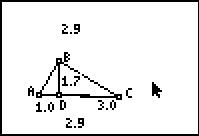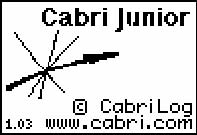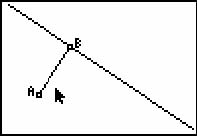••• ##### Device
• TI-83 Plus Family
• TI-84 Plus
• TI-84 Plus Silver Edition
•TI-84 Plus C Silver Edition
•TI-84 Plus CE
• ##### Software

TI Connect™
TI Connect™ CE

# Geometry: The Geometric Mean

by Texas Instruments#### Overview

In this activity, students will establish that several triangles are similar and then determine that the altitude to the hypotenuse of a right triangle is the geometric mean between the segments into which it divides the hypotenuse.

#### Key Steps

•In Problem 1, students use the Cabri™ Jr. App to construct a right triangle with an altitude from the right angle to the hypotenuse. Then, they measure the altitude and the segments of the hypotenuse.

•Students calculate the geometric mean by using the Text and Calculate tools to find the product of the two segments and the square of the altitude. They drag a vertex of the triangle and observe the changes.

•Students change their figure by dragging the vertices. They use the geometric mean of the triangle to estimate the radical values.